You can find the critical values for an ANOVA hypothesis using the F-table. Because the F-distribution is based on two types of degrees of freedom, there's one table for each possible value of alpha (the level of significance).

The following table shows the different values of the F-distribution corresponding to a 0.05 (5 percent) level of significance.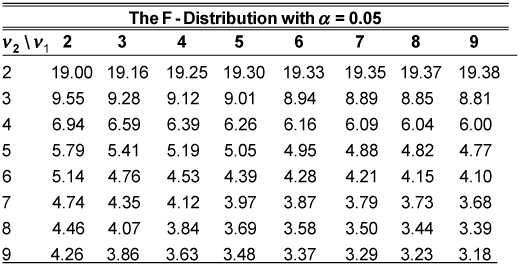The numbers across the top row of the table represent the numerator degrees of freedom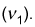You read across this top row to find the appropriate numerator degrees of freedom. The first column represents the denominator degrees of freedom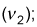you read down this column to find the appropriate denominator degrees of freedom. The critical value is found at the intersection of the row and column you choose. For example, suppose that the numerator degrees of freedom is 5 and the denominator degrees of freedom is 7. The appropriate test statistic is 3.97.

For the one-way ANOVA process, you compute the numerator and denominator degrees of freedom as follows:

• Numerator degrees of freedom = treatments – 1 = t – 1 = 3 – 1 = 2

• Denominator degrees of freedom = total observations minus treatments = N – t = 12 – 3 = 9

For example, say you're looking for a right-tail area of 5 percent under the F-distribution with numerator degrees of freedom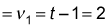and denominator degrees of freedom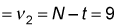You find this critical value at the intersection of the 2 degrees of freedom column and the 9 degrees of freedom row. The critical value equals 4.26, which you can write as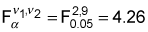The superscripts represent the numerator and denominator degrees of freedom, respectively. The subscript represents the level of significance.